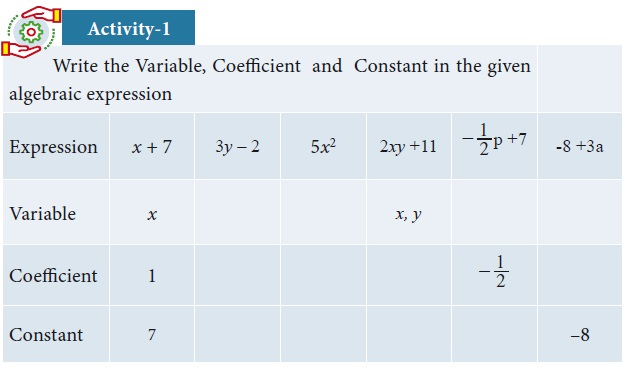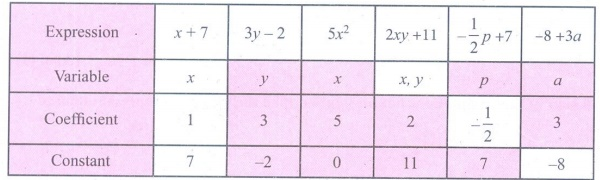Home | | Maths 9th std | Student Activity Questions and Answers

# Student Activity Questions and Answers

Maths : Algebra: Book Back, Exercise, Example Numerical Question with Answers, Solution : Student Activity Questions and AnswersSolution### Activity – 3

1. Find the value of k for the given system of linear equations satisfying the condition below:

(i) 2x + ky = 1; 3x  5y = 7 has a unique solution

(ii) kx + 3= 3; 12+ ky = 6 has no solution

(iii) (k  3)x + 3y = kkx + ky = 12 has infinite number of solution

Solution:2. Find the value of a and b for which the given system of linear equation has infinite number of solutions 3x  (a + 1)y = 2b 1, 5x + (1  2a)y = 3b

Solution:### Activity – 4

For the given linear equations, find another linear equation satisfying each of the given conditionSolution:Tags : Numerical Problems with Answers, Solution | Algebra | Maths , 9th Maths : UNIT 3 : Algebra
Study Material, Lecturing Notes, Assignment, Reference, Wiki description explanation, brief detail
9th Maths : UNIT 3 : Algebra : Student Activity Questions and Answers | Numerical Problems with Answers, Solution | Algebra | Maths